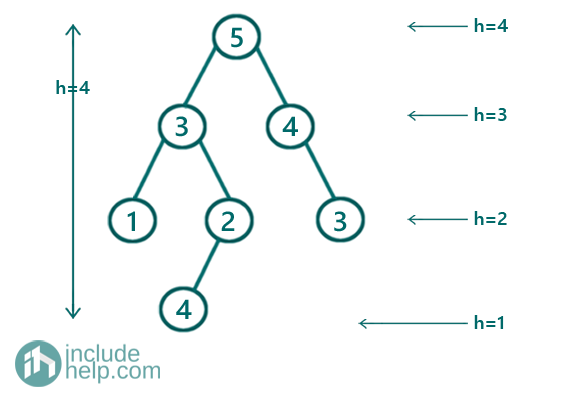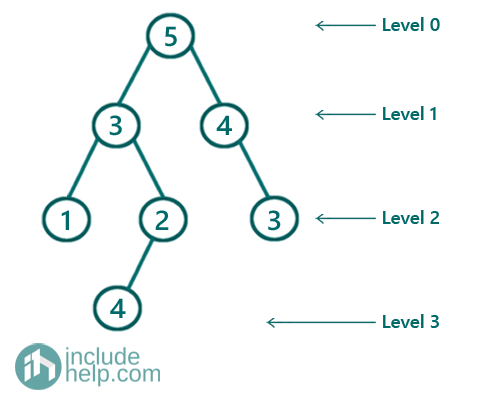# Sum of heights of all the nodes

In this article, we are going to see how we can find sum of heights of all the nodes using level order traversal?
Submitted by Radib Kar, on September 10, 2020

Prerequisite: Level order traversal

Here, we are going to see how we can find sum of heights of all the nodes? For that we have used level order traversal to find the heights.

For example,Here, root has the maximum height of 4
Then node with value 3 & 4 has height 3
Then node with value 1, 2 & 3 has height 2
And finally, node with value 4 has height 1
All heights are from the ground
So total height is 4+ (3+3)+(2+2+2) + 1 = 17

We can solve this using level order traversal. If we do the level order traversal then we will be generated as,So, the difference is here we in level order traversal the heights(levels) are calculated starting from root (root level is 1). So, to transform the heights, we store the levels and later deduct from total height.

For example,

Since level of root is 0 and total height is 4, thus height of root is (4-0)=4

So on.

Below is the implementation for the above idea. Here instead of storing each nodes height, we have stored levels and number of nodes at that level

Using the level order traversal we create the hash table

So for the above example the hash table will be:

Levels Number of nodes at that level
0 1
1 2
2 3
3 1
```1)  To store the sum, initialize a variable sum =0
2)  For level in the hash map:
sum+= (total height – level) * number of nodes at the level
3)  Return sum
```

C++ Implementation:

```#include <bits/stdc++.h>
using namespace std;

// tree node is defined
class TreeNode {
public:
int val;
TreeNode* left;
TreeNode* right;
TreeNode(int data)
{
val = data;
left = NULL;
right = NULL;
}
};

//Find the sum of all heights
int sumOfAllHeights(TreeNode* root)
{
queue<TreeNode*> q;

int sum = 0;

/*
since we are traversing downwards like root is assigned height 0,
we will store number of nodes at height levels,
later we will find original height( total height -level) and
*/

int level = 0, node_at_current_levels = 0;
//first level, root only , level 0
q.push(root);
//end of first level
q.push(NULL);

map<int, int> hash;

//while queue is not empty
while (!q.empty()) {
//DeQueu
TreeNode* temp = q.front();
q.pop();
//if end of current level
if (temp == NULL) {
//if queue is not empty place NULL at the end of
//the queue to denote end of upcoming level
if (!q.empty()) {
q.push(NULL);
}
//store number of nodes at this current level
hash[level] = node_at_current_levels;
//initialize node_at_current_levels to 0 to
//calculate number of nodes for next level
node_at_current_levels = 0;
//increment the level
level++;
}
else {
//increment number of nodes at current level
node_at_current_levels++;
//do level order traversal
if (temp->left)
q.push(temp->left);
if (temp->right)
q.push(temp->right);
}
}

for (auto it = hash.begin(); it != hash.end(); it++) {
sum += (level - it->first) * it->second;
}

return sum;
}

int main()
{
//building the tree like the example
TreeNode* root = new TreeNode(5);

root->left = new TreeNode(3);
root->right = new TreeNode(4);
root->left->left = new TreeNode(1);
root->left->right = new TreeNode(2);
root->right->right = new TreeNode(3);
root->left->right->left = new TreeNode(4);

cout << "Sum of all heights of the nodes is: " << sumOfAllHeights(root) << endl;

return 0;
}
```

Output:

```Sum of all heights of the nodes is: 17
```

What's New

Top Interview Coding Problems/Challenges!

Languages: » C » C++ » C++ STL » Java » Data Structure » C#.Net » Android » Kotlin » SQL
Web Technologies: » PHP » Python » JavaScript » CSS » Ajax » Node.js » Web programming/HTML
Solved programs: » C » C++ » DS » Java » C#
Aptitude que. & ans.: » C » C++ » Java » DBMS
Interview que. & ans.: » C » Embedded C » Java » SEO » HR
CS Subjects: » CS Basics » O.S. » Networks » DBMS » Embedded Systems » Cloud Computing
» Machine learning » CS Organizations » Linux » DOS
More: » Articles » Puzzles » News/Updates